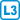## Cycle in a k-connected graph

Prove that in every $$k$$-connected graph any $$k$$ vertices lie on a common cycle.

• #### Hint

Proceed by a contradiction and assume the existence of paths according to Menger’s theorem.

• #### Solution

Let $$S$$ be a given set and $$C$$ be the cycle with as much as possible many vertices from $$S$$.

For a contradiction, assume the existence of $$v \in S \setminus C$$. According to Menger’s theorem, there exist at least $$k$$ disjoint paths between $$v$$ and $$C$$.

The end vertices of these paths divide the cycle $$C$$ into $$k$$ sections. According to Dirichlet’s principle, at least one section does not contain any vertex of $$S$$ inside, therefore, this section can be replaced by the respective two paths leading to $$v$$.

This gives a cycle with more vertices from $$S$$ than $$C$$, which is the desired contradiction.